# Preface

This section demonstrates application of linearization techniwue to modeling of competitions of species.

Introduction to Linear Algebra with Mathematica

# Competing Species

Competition modeling is one of the more challenging aspects of mathematical biology. Competition is clearly important in nature, yet there are so many ways for populations to compete that the modeling is difficult to carry out in any generality. The first general model for competition between n species was proposed by the Russian genius Andrew Kolmogorov in 1936:

$\dot{x}_i = x_i f_i (x_1 , x_2 , \ldots , x_n ) , \qquad I=1,2,\ldots , n.$
His work was a generalization of the paper published by the Russian biologist Georgii Frantsevich Gause in 1934. Since that time, the dynamic systems for modeling of competition have been studied extensively.

Such models reflect the direct impact of one population upon the other. The simplest form of competition, however, occurs when two or more populations compete for the same resource, such as a common food supply or a growth-limiting nutrient. A simple example of this type of competition can be observed in a laboratory device, called a chemostat or a continuous culture, that models competition in a very simple lake. The chemostat is a dynamic system with continuous material inputs and outputs. An ecosystem is so complex, so difficult to comprehend, that any attempt to understand its interactions is frequently doomed to failure because of a luck of rigorous controls. Consequently, in many cases we study population interactions under simplified, controllable laboratory conditions.

In this section, we explore application of two- and higher-dimensional systems of differential equations in some problems of population dynamics. We start with populations of two species that occupy the same habitat and compete for limited resources. Although the equations we start with are extremely simple compared to the very complex relationships that exist in nature, it allows us to get some insights into ecological problems. A number of mathematical models have been constructed to address this issue.

Recall from the first part of the tutorial the logistic equation for a single species:

$\frac{{\text d}N}{{\text d}t} = r\,N \left( 1 - \frac{N}{K} \right) ,$
where r is the growth (birth) rate, and K the carrying capacity. We assume that populations of two species varies continuously and use letters x(t) and y(t) to represent their populations at time t. For these two species living in the same habitat, each of them tends to diminish the available resources for the other. In effect, they reduce each other's growth rates and saturation populations. The simplest model for reducing growth rate of species, say x, due to the presence of species y is toe replace the growth rate factor (1 - N/K) in the logistic equation by the following expressions:
$\begin{split} \dot{x} &= x \left( r_1 - a_1 x - b_1 y \right) , \\ \dot{y} &= y \left( r_2 - a_2 x - b_2 y \right) , \end{split}$
where dot stands for the derivative with respect to time variable t. Here ri is the maximum per capita growth of species i, a1 and b1 represent the absolute intra-and inter-specific competition coefficients, respectively. Sometimes, the above competition equations can be reduced to a dimensionless case by introducing a new time variable τ = r1t and set r = r2/r1. Upon setting y1 = xb1, y2 = yb2, and a12 = b1r2/(a2r1), a21 = b2r1/(a1r2), we get the system of equations with fewer parameters:
$\begin{split} \dot{y}_1 &= y_1 \left( 1 - y_1 - a_{12} y_2 \right) , \\ \dot{y}_2 &= r\,y_2 \left( 1 - y_2 - a_{21} y_1 \right) . \end{split}$
To find critical points, we need to solve the system of equations
$x \left( r_1 - a_1 x - b_1 y \right) =0, \qquad y \left( r_2 - a_2 x - b_2 y \right) =0$
that locate the nullclines. There are three obvious extinguishing critical points
$O(0,0), \qquad A\left( 0,r_2 / a_2 \right) , \qquad B\left( r_1 / a_1 , 0 \right) ,$
and the fourth at the intersection of these two lines
$\begin{cases} r_1 &= a_1 x + b_1 y, \\ r_2 &= a_2 x + b_2 y . \end{cases}$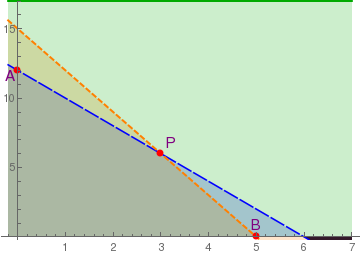p = Plot[{12 - 2*x, 15 - 3*x, 17}, {x, -0.2, 7}, PlotRange -> {-0.1, 17}, PlotStyle -> {{Thick, Dashed, Orange}, {Darker@Green, Thick}}, Filling -> Axis, PlotRange->{0,15} FillingStyle -> Automatic] p1 = Graphics[{Red, PointSize[Large], Point[{3,6}]}]; p2 = Graphics[{Red, PointSize[Large], Point[{0,12}]}]; p3 = Graphics[{Red, PointSize[Large], Point[{5,0}]}]; t1 = Graphics[Text[Style["A", FontSize -> 14, Purple], {-0.16, 11.6}]]; t2 = Graphics[Text[Style["B", FontSize -> 14, Purple], {5, 0.9}]]; t3 = Graphics[Text[Style["P", FontSize -> 14, Purple], {3.2, 6.8}]]; Show[p,p1,p2,p3, t1,t2,t3, AspectRatio->2/3] Critical points. Mathematica code

Example: We demonstrate how Mathematica can be helpful with plotting domains related to competition of species. First, we just plot the nullclines.

Plot[{12 - 2 x, 15 - 3 x}, {x, 0, 7}, PlotRange -> {{0, 7}, {0, 17}}]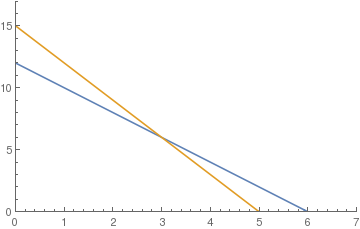The same graph using Filling option
Plot[{12 - 2 x, 15 - 3 x}, {x, 0, 7}, PlotRange -> {{0, 7}, {0, 17}}, Filling -> Axis]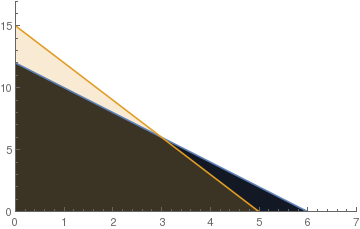Another version:
Plot[{12 - 2 x, 15 - 3 x}, {x, 0, 7}, PlotRange -> {{0, 7}, {0, 17}}, Filling -> {1 -> {{2}, {Yellow, Green}}}]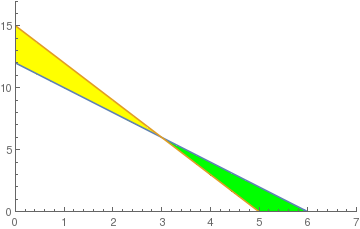Adding few extra lines for our usage
Plot[{12 - 2 x, 15 - 3 x, -1, 18}, {x, 0, 7},
PlotRange -> {{0, 7}, {0, 17}}, Filling -> {1 -> {{2}, {Directive[Opacity[0.5], Yellow],
Directive[Opacity[0.5], Green]}}}, Axes -> False, Frame -> True]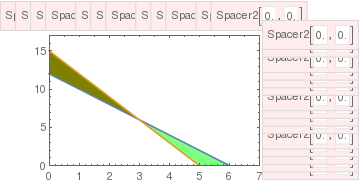Plotting with full domain:
Plot[{12 - 2 x, 15 - 3 x, -1, 18}, {x, 0, 7},
PlotRange -> {{0, 7}, {0, 17}}, Filling -> { 1 -> {{2}, {Directive[Opacity[0.5], Yellow],
Directive[Opacity[0.5], Green]}}, 2 -> {{3}, {Directive[Opacity[0.5], RandomColor[]],
Directive[Opacity[0.5], RandomColor[]]}}, 4 -> {{2}, {Directive[Opacity[0.5], RandomColor[]],
Directive[Opacity[0.5], RandomColor[]]}}}, Axes -> False, Frame -> True]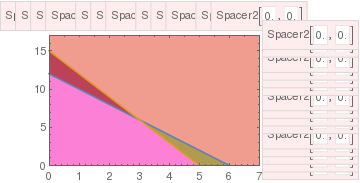If you prefer to remove ticks you can do as follows:
Plot[{12 - 2 x, 15 - 3 x, -1, 18}, {x, 0, 7},
PlotRange -> {{0, 7}, {0, 17}}, Filling -> { 1 -> {{2}, {Directive[Opacity[0.5], Yellow],
Directive[Opacity[0.5], Green]}},
2 -> {{3}, {Directive[Opacity[0.5], RandomColor[]]}},
4 -> {{2}, {Directive[Opacity[0.5], RandomColor[]]}}},
Axes -> False, Frame -> True, FrameTicks -> None]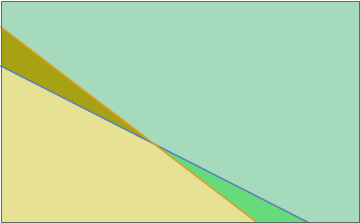The only feasible (non-negative populations!) can occur at point
$P \left( \frac{a_2 r_1 - b_1 r_2}{a_1 a_2 - b_1 b_2} , \frac{a_1 r_2 - b_2 r_1}{a_1 a_2 - b_1 b_2} \right) = P \left( \frac{1- a_{12}}{1-a_{12}a_{21}} , \frac{1- a_{21}}{1-a_{12}a_{21}} \right) , \qquad a_{12} = \frac{b_1 r_2}{a_2 r_1} , \quad a_{21} = \frac{b_2 r_1}{a_1 r_2} ,$
when
$\begin{split} a_2 r_1 - b_1 r_2 &> 0 , \\ a_1 r_2 - b_2 r_1 &> 0 , \end{split} \qquad\qquad\mbox{or} \qquad a_{12} <1 , \quad a_{21} <1 .$
Note that we do not consider critical points outside the first quadrant because they have no biological meaning (population cannot be negative). If these two lines do not cross inside the first quadrant ($$x, y \ge 0$$ ), then there are only three equilibria on its boundary. In this case, the two species cannot coexist in peaceful equilibrium, and at least one of them will die out. This case is referred to as the competitive exclusion principle, Gause's law of competitive exclusion, or just Gause's law because it was originally formulated by Georgii Gause in 1934 on the basis of experimental evidence. It states that two species competing for the same resources cannot coexist if other ecological factors are constant. For instance, a competition in the 20th century between the USA and USSR resulted in the inevitable defeat of the latter.

Now we analyze stability of the given model using a linearization technique. We consider each equilibrium solution separately based on the properties of the corresponding Jacobian matrix:

$J(x,y) = \begin{bmatrix} r_1 -2a_1 x - b_1 y & -b_1 x \\ -b_2 y & r_2 - 2a_2 y - b_2 x \end{bmatrix} .$
1. The origin (0,0) is unstable node because its Jacobian
$J(0,0) = \begin{bmatrix} r_1 & 0 \\ 0 & r_2 \end{bmatrix} .$
has two positive eigenvalues.
2. Point A( r1/a1 , 0) could be unstable (a1r2 > b2r1) or stable (a1r2 < b2r1) because its Jacobian at this point
$J(A) = J\left( \frac{r_1}{a_1} , 0 \right) = \begin{bmatrix} -r_1 & -b_1 r_1 / a_1 \\ 0 & \left( a_1 r_2 - b_2 r_1 \right) /a_1 \end{bmatrix}$
has one negative eigenvalue $$\lambda_1 = -r_1$$ and another one $$\lambda_2 = \left( a_1 r_2 - b_2 r_1 \right) / a_1 .$$
3. Point B( 0, r2/a2) could be unstable (a1r2 > b2r1) or stable (a1r2 < b2r1) because its Jacobian at this point
$J(B) = J\left( 0 , \frac{r_2}{a_2} \right) = \begin{bmatrix} \left( r_1 a_2 - b_1 r_2 \right) / a_2 & 0 \\ - b_2 r_2 / a_2 & - r_2 \end{bmatrix}$
has one negative eigenvalue $$\lambda_1 = -r_2$$ and another one $$\lambda_2 = \left( a_2 r_1 - b_1 r_2 \right) / a_2 .$$
4. The Jacobian at point P has real eigenvalues; their signs depend on possible sign combinations of numerators and denominators.
There are two cases when point P is in the first quadrant (for coexistence of two competitors):
1. a12 < 1 and a21 < 1, which is equivalent to
$\frac{b_2}{a_1} < \frac{r_2}{r_1} < \frac{a_2}{b_1} \qquad\mbox{and} \qquad a_1 a_2 - b_1 b_2 >0.$
The point P is asymptotically stable. All other critical points are unstable. In terms of the ecology, this means that the interspecific competition is not too strong the two populations can coexist stably, but at lower populations than their respective carrying capacities. Thus, although the species may coexist, the price that they pay for competing with each other is that they do not reach the population density that they would have achieved (i.e. their carrying capacity) with the other species absent.
2. a12 > 1 and a21 < 1, which is equivalent to
$\frac{a_2}{b_1} < \frac{r_2}{r_1} < \frac{b_2}{a_1} \qquad\mbox{and} \qquad a_1 a_2 - b_1 b_2 <0.$
The critical point P is a saddle point (unstable). Points A and B on the boundary are asymptotically stable nodes. Therefore, in this case one of the populations extincts. This means that interspecific competition is aggressive and ultimately one population wins, while the other is driven to extinction. The winner depends upon which has the starting advantage.

Example: Consider the following model that describes the competition between two species:

$\begin{split} \dot{x} &= x \left( 19 -3x-y \right) , \\ \dot{y} &= y \left( 13 -x-2y \right) . \end{split}$
As Mathematica shows
Solve[{x*(19-3*x -y) == 0, y*(13-x -2*y) == 0}, {x,y}]
critical points occur at
$O(0,0), \qquad A\left( 0, \frac{13}{2} \right) , \qquad B \left( \frac{19}{3} , 0 \right) , \qquad P(5,4) .$
Then we plot the phase portrait for the given system of differential equations
StreamPlot[{x*(19-3*x -y), y*(13-x -2*y)}, {x, -2, 7}, {y, -2, 8}, StreamColorFunction -> "Rainbow"]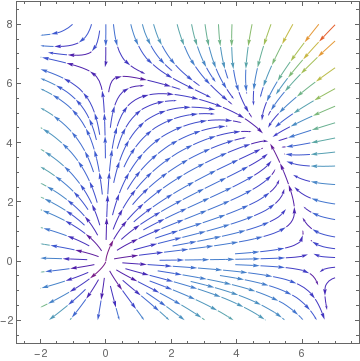The Jacobian matrix is
${\bf J}(x,y) = \begin{bmatrix} 19-6x-y & -x \\ -y & 13 -x -4y \end{bmatrix} .$
Then at critical points, we have
${\bf J}(0,0) = \begin{bmatrix} 19 & 0 \\ 0 & 13 \end{bmatrix} , \quad {\bf J}(A) = \begin{bmatrix} \frac{25}{2} & 0 \\ -\frac{13}{2} & -13 \end{bmatrix} , \quad {\bf J}(B) = \begin{bmatrix} -19&-\frac{19}{3} \\ 0 & \frac{20}{3} \end{bmatrix} , \quad {\bf J}(P) = \begin{bmatrix} -15&-5 \\ -4&-8 \end{bmatrix} .$
Since the eigenvalues of J(O) 19 and 13, the origin is an unstable node. Both points A and B are unstable saddle points because the their Jacobian matrices has one positive eigenvalue and one negative eigenvalue. The eigenvalues of matrix J(P) are
j[x_, y_] = {{19 - 6*x - y, -x}, {-y, 13 - x - 4*y}}
Eigenvalues[j[5,4]]
$\lambda = - \frac{23}{2} \pm \frac{\sqrt{129}}{2} \qquad \mbox{or} \qquad -17.1789 , \quad -5.82109$
both negative, so point P is an asymptotically stable node. ■

Example: Consider the following model:

$\begin{split} \dot{x} &= x \left( 3 -2x-3y \right) , \\ \dot{y} &= y \left( 5 -4x-4y \right) . \end{split}$
This system of differential equations has four real stationary points:
$O(0,0), \qquad A\left( 0, \frac{5}{4} \right) , \qquad B \left( \frac{3}{2} , 0 \right) , \qquad P\left( \frac{3}{4} , \frac{1}{2} \right) .$
StreamPlot[{3*x-2*x*x-3*x*y, 5*y-4*x*y-4*y*y}, {x,-0.5,3},{y,-0.5,3}, StreamStyle->Yellow, FrameStyle->LightGray, Background->Black]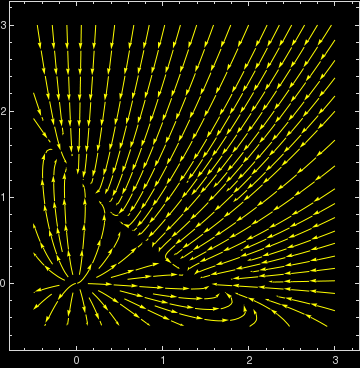The numerical values of general parameters are
$r_1 =3, \quad a_1 =2 , \quad b_1 =3 , \qquad r_2 =5, \quad a_2 =4, \quad b_2 =4 .$
we have
$\frac{a_2}{b_1} = \frac{4}{3} < \frac{r_2}{r_1} = \frac{5}{3} < \frac{b_2}{a_1} = \frac{4}{2} =2 \quad\mbox{and} \quad a_1 a_2 -b_1 b_2 = 8-12<0.$
Also
$a_{12} = \frac{b_1 r_2}{a_2 r_1} = \frac{5}{4} >1 \qquad\mbox{and}\qquad a_{21} = \frac{b_2 r_1}{a_1 r_2} = \frac{6}{5} >1.$
The Jacobian
${\bf J}(x,y) = \begin{bmatrix} 3-4x-3y & -3x \\ -4y & 5 -4x -8y \end{bmatrix} .$
at critical points is
${\bf J}(0,0) = \begin{bmatrix} 3 & 0 \\ 0 & 5 \end{bmatrix} , \quad {\bf J}(A) = \begin{bmatrix} -\frac{3}{4} & 0 \\ -\frac{13}{2} & -13 \end{bmatrix} , \quad {\bf J}(B) = \begin{bmatrix} -3&-\frac{9}{2} \\ 0 & -1 \end{bmatrix} , \quad {\bf J}(P) = \begin{bmatrix} -\frac{3}{2}&-\frac{9}{4} \\ -2&-2 \end{bmatrix} .$
Obviously, the origin is unstable node because the linearized matrix has two positive eigenvalues. The eigenvalues of the Jacobian matrix at other critical points are
j[x_,y_]= {{3-4*x-3*y,-3*x},{-4*y,5-4*x-8*y}}
Eigenvalues[j[0,0]]
{5, 3}
Eigenvalues[j[0,5/4]]
{-5, -(3/4)}
Eigenvalues[j[3/2,0]]
{-3, -1}
Eigenvalues[j[3/4,1/2]]
{1/4 (-7 - Sqrt), 1/4 (-7 + Sqrt)}
So we see that stationary points A and B are asymptotically stable, but the critical point P is unstable saddle point. ■

Example: Consider competition of two species modeled by the system

$\begin{split} \dot{x} &= x \left( 3 -2x-y \right) , \\ \dot{y} &= y \left( 1 -3x-y/4 \right) . \end{split}$
With the aid of Mathematica, we find all four critical points:
Solve[{x*(3 - 2*x - y) == 0, y*(1 - 3*x - y/4) == 0}, {x, y}]
{{x -> 0, y -> 4}, {x -> 1/10, y -> 14/5}, {x -> 3/2, y -> 0}, {x -> 0, y -> 0}}
Then we plot the phase portrait.
StreamPlot[{3*x-2*x*x-x*y, y-3*x*y-y*y/4}, {x,-0.5,3},{y,-1,5}, Mesh -> {{{2, Thick}, {4, Green}, {5, {Thick, Red, Dashed}}}}]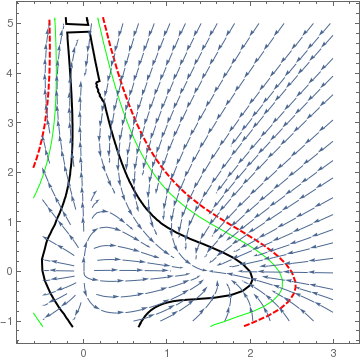According to linearization approach, we need to evaluate the Jacobian
${\bf J}(x,y) = \begin{bmatrix} 3-4x-y & -x \\ -3y & 1 -3x -y/2 \end{bmatrix} .$
at every stationary point
$O(0,0), \qquad A\left( 0, 4 \right) , \qquad B \left( \frac{3}{2} , 0 \right) , \qquad P\left( \frac{1}{10} , \frac{14}{5} \right) .$
Mathematica helps
j[x_,y_]= {{3-4*x-y,-x},{-3*y,1-3*x-y/2}}
Eigenvalues[j[0,0]]
{3, 1}
Eigenvalues[j[0,4]]
{-1, -1}
Eigenvalues[j[3/2,0]]
{-(7/2), -3}
Eigenvalues[j[1/10,14/5]]
{-(7/5), 1/2}
Since eigenvalues at points A and B are all negative, these points are asymptotically stable nodes. On the other hand, point P is a saddle point, so it is unstable. ■

Example: Consider a model that describes the competition between two species as

$\begin{split} \dot{x} &= x \left( 3 -2x-3y \right) , \\ \dot{y} &= y \left( 6 -4x-2y \right) . \end{split}$
Its critical points are
Solve[{x*(3 - 2*x - 3*y) == 0, y*(6 - 4*x - 2*y) == 0}, {x, y}]
{{x -> 0, y -> 3}, {x -> 3/2, y -> 0}, {x -> 0, y -> 0}}
So this system has only three stationary points, and all of them are on the boundary. This means that one of populations will extinguish eventually. According to given numerical values, we have
$a_{12} = \frac{b_1 r_2}{a_2 r_1} = \frac{3\cdot 3}{2\cdot 6} = \frac{3}{4} <1 \qquad \mbox{and} \qquad a_{21} = \frac{b_2 r_1}{a_1 r_2} = \frac{4\cdot 6}{2\cdot 3} = 4>1.$
Based on the Jacobian
j[x_,y_]= {{3-4*x-3*y,-3*x},{-4*y,6-4*x-4*y}}
${\bf J}(x,y) = \begin{bmatrix} 3-4x-3y & -3x \\ -4y & 6 -4x -4y \end{bmatrix} ,$
we evaluate it at every critical point:
${\bf J}(0,0) = \begin{bmatrix} 3 & 0 \\ 0 & 6 \end{bmatrix} , \quad {\bf J}(0,3) = \begin{bmatrix} -6 & 0 \\ 0 & -6 \end{bmatrix} , \quad {\bf J}(3/2,0) = \begin{bmatrix} -3 & 0 \\ 0 & 0 \end{bmatrix} .$
Therefore, the origin is unstable node, but point A(0,3) is an improper nodal sink (asymptotically stable). We cannot analyze point (3/2,0) using the linearization method because it is not isolated (one eigenvalue is zero). So we make another more closer look at its phase portrait.
StreamPlot[{3*x-2*x*x-3*x*y, 6*y-4*x*y-2*y*y}, {x,-1,3},{y,-1,5}, MeshFunctions -> Function[{x,y,vx,vy,n},n], Mesh->15]
StreamPlot[{3*x - 2*x*x - 3*x*y, 6*y - 4*x*y - 2*y*y}, {x, -0.5, 0.5}, {y, 2.5, 3.5}, StreamStyle -> LightGray, VectorPoints ->8, PlotRange ->All, VectorStyle -> Graphics[Circle[]], VectorScale -> {Automatic, Scaled[0.3]}]
StreamPlot[{3*x - 2*x*x - 3*x*y, 6*y - 4*x*y - 2*y*y}, {x, 1, 2}, {y, -0.5, 0.5}, Mesh -> 10, MeshShading -> {Red, Yellow, Green, None}]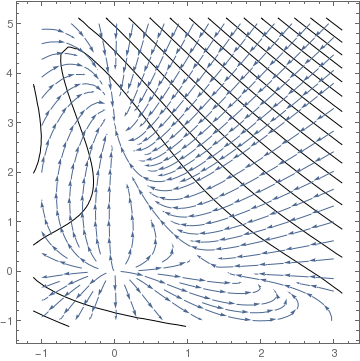Phase portrait of the given system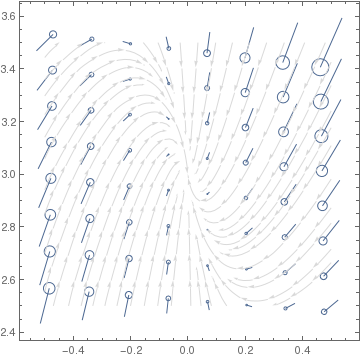Phase portrait near critical point (0,3)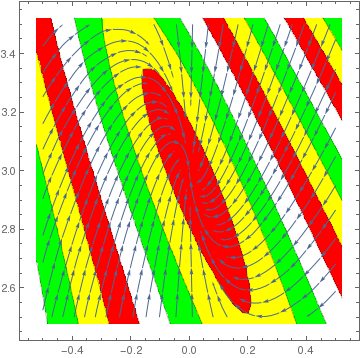Phase portrait near critical point (1.5,0)
Therefore, we conclude that, based on phase portrait, the stationary point (3/2,0) is asymptotically stable. ■

Example: Consider the following asymmetric May--Leonard model:

$\begin{split} \dot{x}_1 = x_1 \left( 1 - x_1 - \alpha_1 x_2 - \beta_1 x_3 \right) , \\ \dot{x}_2 = x_2 \left( 1 - \beta x_1 - x_2 - \alpha_2 x_3 \right) , \\ \dot{x}_3 = x_3 \left( 1 - \alpha_3 x_1 - \beta_3 x_2 - x_3 \right) , \end{split}$
under the assumptions
$0 < \alpha_i < 1 < \beta_i , \qquad i=1,2,3.$
The above system models the competition between three species with the same intrinsic growth rates and different competition coefficients.
Theorem: The above system of differential equations under mentioned constraint has a unique interior equilibrium
$P = (p_1 , p_2 , p_3 ) = \left( \frac{\Delta_1}{\Delta} , \frac{\Delta_2}{\Delta} , \frac{\Delta_3}{\Delta} \right) ,$
where
$\begin{split} \Delta_1 &= A_1 A_2 + A_2 B_3 + B_3 B_1 , \\ \Delta_2 &= A_2 A_3 + A_3 B_1 + B_1 B_2 >0 , \\ \Delta_3 &= A_3 A_1 + A_1 B_2 + B_2 B_3 >0, \end{split}$
with
$A_i = 1-\alpha_i >0, \quad B_i = \beta_i -1 >0, \qquad i=1,2,3.$

1. Two species models
2. Chi C-W, Hsu S-B, and Wu L-I., On the asymmetric May--Leonard model of three competing species, SIAM Journal on Applied Mathematics, 58, No 1, 1998, 211--226.
3. May R M and Leonard W J, Nonlinear aspects of competition between three species, SIAM Journal on Applied Mathematics, 29, No 2, 1975, 243--253. https://doi.org/10.1137/0129022
4. McGehee Richard and Armstrong Robert A., Some mathematical problems concerning the ecological principle of competitive exclusion, Journal of Differential Equations, 23, No 1, 1977, 30--52. doi: 10.1016/0022-0396(77)90135-8